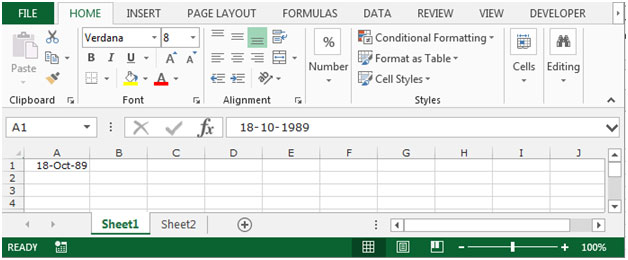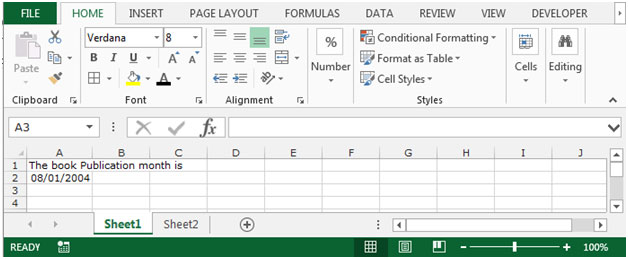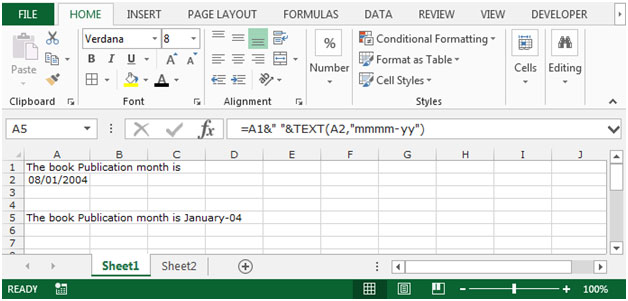# Combining Text and a Formatted Date in Excel 2010

Sometimes when we prepare analysis reports, we show the date with text and if we want to make any changes in data it should be updated automatically in analysis points in Excel 2010.

To do this we use the “&” symbol in our function. This symbol is used to add the text with formatted date.

Let’s take an example to understand that how we used the “&” symbol with calculated numbers.

We have a date of birth in cell A1, we want to calculate the age till today. We want age to be automatically calculated on a daily basis. Also age should appear with the text years in cell B1.

To calculate the age we use the Today formula along with Round formula, in which today formula will work for today’s date and Round formula will round the figure which we got after the calculation of age.To do this follow below given steps:-

• Select the cell B1 and write the formula.
• =ROUND((TODAY()-A1)/365,0)&" Yrs", press enter.
• This function will return age in this “25 Yrs” format.

Let’s understand with another example, how to excel combine text and formatted date.

We have text and formulated value in the range A1:A2. In which A1 is containing the text and cell A2 is containing the date.If you want to merge two text cells and date, follow below mentioned steps:-

• Select the cell A5, and write the formula.
• Equals to select the A2 type the sign of “&” space in between inverted commas, again type the sign of “&” type the function name “text” bracket open, select the cell A2 (date) comma type the text format as per your requirement I will type the format here “MMMM-YY” bracket close press enter.
• =A1&" "&TEXT(A2,"mmmm-yy")Note: If the any changes will update in text and date, the statement will get changed automatically.

This is how we can join two text in excel with formatted date in Microsoft Excel 2010 and 2013.

## Users are saying about us...

1.I HAVE BEEN TRYING TO INTEGRATE A FORM WITH A EXCEL SPREADSHEET SO THAT INFORMATION IS PUT IN THE FORM WHICH AUTOMATICALLY UPDATES THE SPREAD SHEET BUT IT DOESNT WORK

2.I HAVE BEEN TRYING TO INTEGRATE A FORM WITH A EXCEL SPREADSHEET SO THAT INFORMATION IS PUT IN THE FORM WHICH AUTOMATICALLY UPDATES THE SPREAD SHEET BUT IT DOESNT WORK

Terms and Conditions of use

The applications/code on this site are distributed as is and without warranties or liability. In no event shall the owner of the copyrights, or the authors of the applications/code be liable for any loss of profit, any problems or any damage resulting from the use or evaluation of the applications/code.